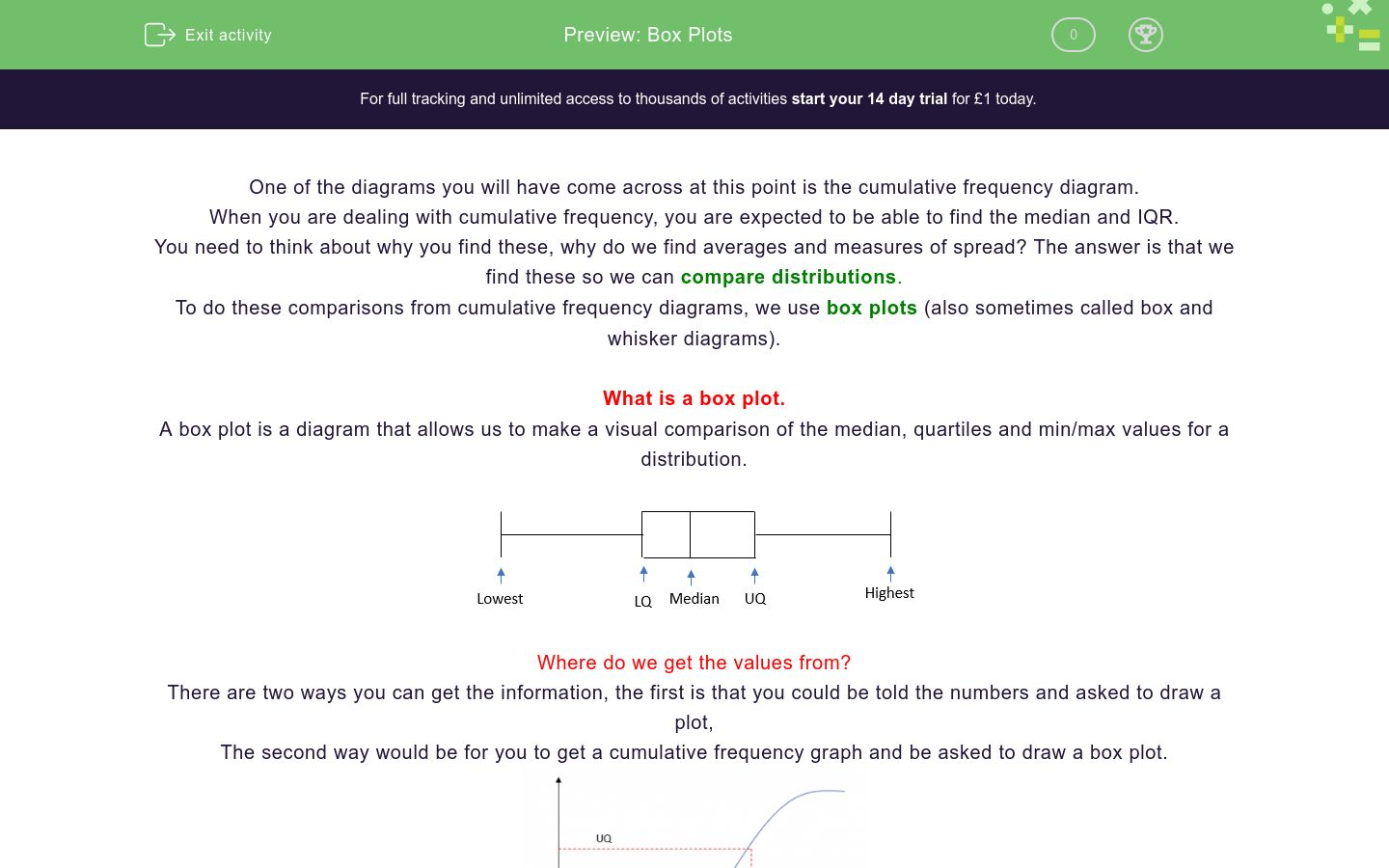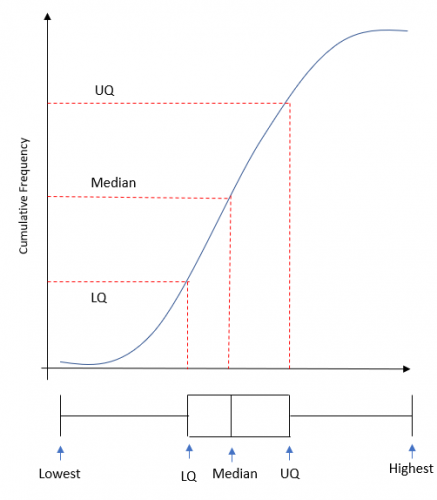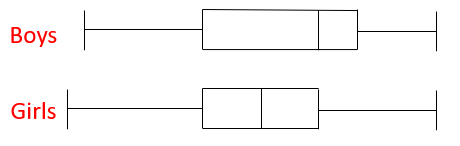# Box Plots

In this worksheet, students practise reading and constructing box plots.Key stage:  KS 4

GCSE Subjects:   Maths

GCSE Boards:   AQA, Eduqas, Pearson Edexcel, OCR

Curriculum topic:   Statistics

Curriculum subtopic:   Statistics, Interpreting and Representing Data

Difficulty level:### QUESTION 1 of 10

One of the diagrams you will have come across at this point is the cumulative frequency diagram.

When you are dealing with cumulative frequency, you are expected to be able to find the median and IQR.

You need to think about why you find these, why do we find averages and measures of spread? The answer is that we find these so we can compare distributions.

To do these comparisons from cumulative frequency diagrams, we use box plots (also sometimes called box and whisker diagrams).

What is a box plot.

A box plot is a diagram that allows us to make a visual comparison of the median, quartiles and min/max values for a distribution.Where do we get the values from?

There are two ways you can get the information, the first is that you could be told the numbers and asked to draw a plot,

The second way would be for you to get a cumulative frequency graph and be asked to draw a box plot.If any of the information cannot be read from the graph (this is sometimes the case for the highest/lowest), you will be told them in the question.

Box plots are used to....

Which of these box plots have been drawn correctly?A

B

C

A distribution has a lowest value of 10, a highest value of 19, a median of 14.5 and an IQR of 3.

Which of these diagrams have been drawn correctly?A

B

C

D

What is the median for this box plot?What is the Inter Quartile Range for this box plot?The heights of a group of boys and girls are measured and box plots are produced.Are boys or girls taller on average?

Boys

Girls

The heights of a group of boys and girls are measured and box plots are produced.Which groups heights were more consistent?

Boys

Girls

I have some some data that is in a grouped frequency diagram.

If I create a cumulative frequency diagram and then a box plot. Which of the five features of a box plot will I be able to find?

Lowest value

LQ

Median

UQ

Highest

For this cumulative frequency diagram, find the median, UQ and LQLowest value

LQ

Median

UQ

Highest

For this cumulative frequency diagram, find the median, UQ and LQLowest value

LQ

Median

UQ

Highest

• Question 1

Box plots are used to....

EDDIE SAYS
There's only one thing you would use these for and that is to compare the distributions.
• Question 2

Which of these box plots have been drawn correctly?B
EDDIE SAYS
Its really important to draw these correctly, there's actually a mark for doing it in the exam. The horizontal line should not go all the way across There should be exactly 5 vertical lines.
• Question 3

A distribution has a lowest value of 10, a highest value of 19, a median of 14.5 and an IQR of 3.

Which of these diagrams have been drawn correctly?C
EDDIE SAYS
This is a bit of a process of elimination. The first fact is that the lowest value is 10, this eliminates A immediately. The next fact that matters is that the median is 14.5, this eliminates another, keep doing this until you get the answer.
• Question 4

What is the median for this box plot?16
EDDIE SAYS
This is just testing if you know which one the median is. Read of the value from the line in the middle of the box.
• Question 5

What is the Inter Quartile Range for this box plot?3
EDDIE SAYS
IQR = UQ - LQ The upper quartile is the right-hand side of the box The lower quartile is the left-hand side of the box Read off the values and work out your IQR
• Question 6

The heights of a group of boys and girls are measured and box plots are produced.Are boys or girls taller on average?

Boys
EDDIE SAYS
For this, we need to look at the medians, the boys one is higher than the girls so on average...
• Question 7

The heights of a group of boys and girls are measured and box plots are produced.Which groups heights were more consistent?

Girls
EDDIE SAYS
Consistency is linked to the IQR. The smaller the IQR, the more consistent the data. Which one of the groups has the smaller IQR?
• Question 8

I have some some data that is in a grouped frequency diagram.

If I create a cumulative frequency diagram and then a box plot. Which of the five features of a box plot will I be able to find?

LQ
Median
UQ
EDDIE SAYS
When you have grouped data, you don't know what the lowest and highest values are, only what groups they are in. For this question, they would tell you the lowest and highest value.
• Question 9

For this cumulative frequency diagram, find the median, UQ and LQEDDIE SAYS
We have 30 pieces of data To find the median, we go across from 15 and read off the value on the bottom scale. To find the UQ and LQ, we do the same from the 22.5th and 7.5th values. Remember that you will get a margin of error as you are reading from a graph.
• Question 10

For this cumulative frequency diagram, find the median, UQ and LQEDDIE SAYS
We have 50 pieces of data To find the median, we go across from 25 and read off the value on the bottom scale. To find the UQ and LQ, we do the same from the 37.5th and 12.5th values. Remember that you will get a margin of error as you are reading from a graph.
---- OR ----

Sign up for a £1 trial so you can track and measure your child's progress on this activity.

### What is EdPlace?

We're your National Curriculum aligned online education content provider helping each child succeed in English, maths and science from year 1 to GCSE. With an EdPlace account you’ll be able to track and measure progress, helping each child achieve their best. We build confidence and attainment by personalising each child’s learning at a level that suits them.

Get started